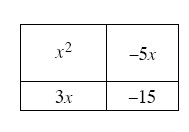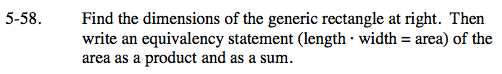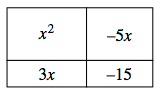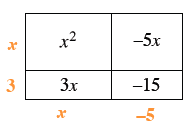Home > CAAC > Chapter 5 > Lesson 5.1.6 > Problem5-58

5-58.

Find the dimensions of the generic rectangle below. Then write an equivalency statement (length · width = area) of the area as a product and as a sum. Homework Help ✎First, find the dimensions of the rectangle.
(x + 3) and (x − 5)

Area = (Length)(Width)

(x + 3)(x − 5) = x2 − 2x − 15